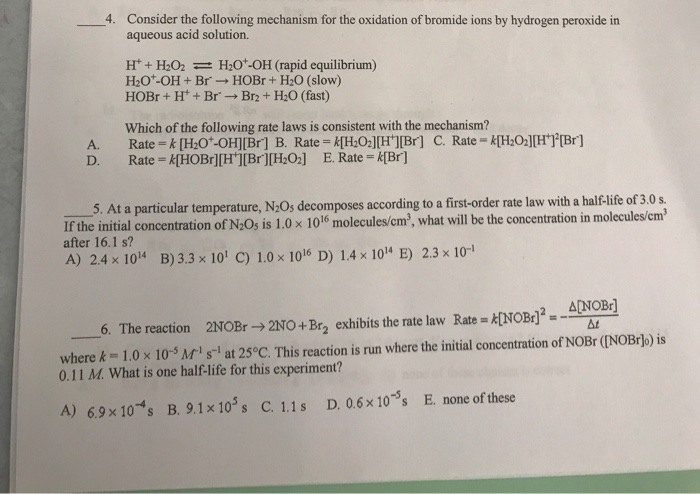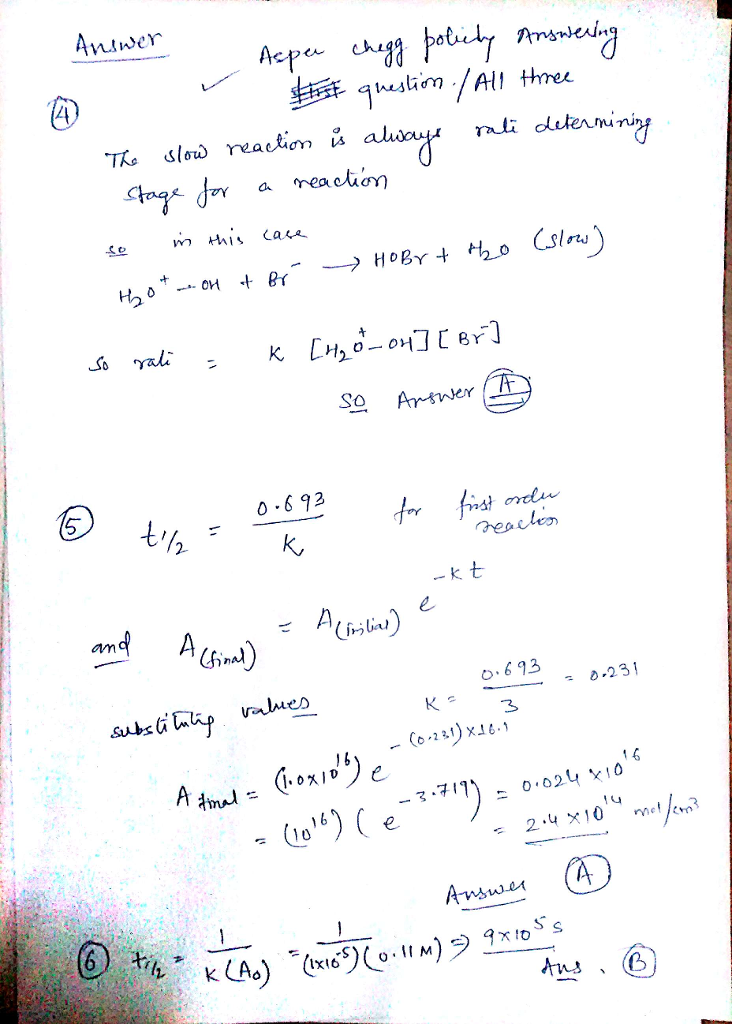# Question & Answer: Please answer but also explain all of them! Thank you! Consider the following mechanism for the oxidation…..Please answer but also explain all of them! Thank you!

Consider the following mechanism for the oxidation of bromide ions by hydrogen peroxide in aqueous acid solution. H^+ + H_2O_2 H_2O^+-OH (rapid equilibrium) H_2O^+-OH + Br^- rightarrow HOBr + H_2O (slow) HOBr + H^+ + Br^- rightarrow Br_2 + H_2O (fast) Which of the following rate laws is consistent with the mechanism? A. Rate = k[H_2O^+ – OH][Br^-] B. Rate = k[H_2O_2][H^+][Br^] C. Rate = k[H_2O_2][H^+]^2[Br^] D. Rate k[HOBr][H^+][Br^-][H_2O_2] E. Rate K[Br^] At a particular temperature, N_2O_5 decomposes according to a first-order rate law with a half-life of 3.0 s. If the initial concentration of N_2O_5 is 1.0 times 10^16 molecules/cm^3, what will be the concentration in molecules/cm^3 after 16.1 s? A) 2.4 times 10^14 B) 3.3 times 10^1 C) 1.0 times 10^16 D) 1.4 times 10^14 E) 2.3 times 10^-1 The reaction 2NOBr rightarrow 2NO + Br_2 exhibits the rate law Rate = k [NOBr]^2 = – Delta [NOBr]/Delta t where k = 1.0 times 10^-5 M^-1 s^-1 at 25 degree C. This reaction is run where the initial concentration of NOBr ([NOBr]o) is 0.11 M. What is one half-life for this experiment? A) 6.98 times 10^-4 s B.9. 1 times 10^5 s C. 1.1 s D. 0.6 times 10^-5s E. none of these